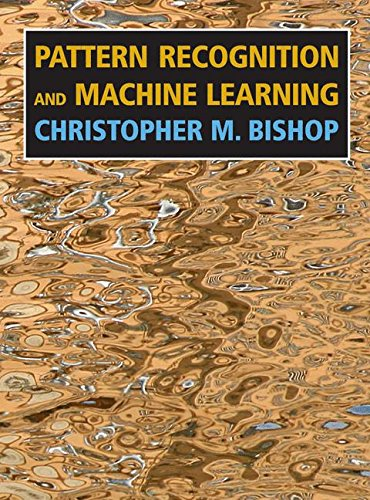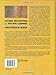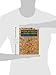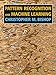Tuesday , May 26th 2020

# Pattern Recognition and Machine Learning (Information Science and Statistics)#### Product Features:

This is the first textbook on pattern recognition to present the Bayesian viewpoint. The book presents approximate inference algorithms that permit fast approximate answers in situations where exact answers are not feasible. It uses graphical models to describe probability distributions when no other books apply graphical models to machine…
Price as on: 2019-12-22 14:11:33

#### Product Description

This is the first textbook on pattern recognition to present the Bayesian viewpoint. The book presents approximate inference algorithms that permit fast approximate answers in situations where exact answers are not feasible. It uses graphical models to describe probability distributions when no other books apply graphical models to machine learning. No previous knowledge of pattern recognition or machine learning concepts is assumed. Familiarity with multivariate calculus and basic linear algebra is required, and some experience in the use of probabilities would be helpful though not essential as the book includes a self-contained introduction to basic probability theory.

## Complexity Theory, Game Theory, and Economics: The

This monograph comprises a series of ten lectures divided into two parts. Part 1, referred to as the Solar…

## Computer Science Education

This book provides an overview of how to approach computer science education research from a pragmatic perspective. It represents…

## Supervised Learning with Quantum Computers (Quantum

Quantum machine learning investigates how quantum computers can be used for data-driven prediction and decision making. The books summarises…